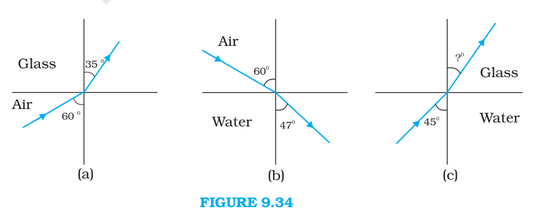# Q 9.4    Figures of (a) and (b) show refraction of a ray in air incident at  with the normal to a glass-air and water-air interface, respectively. Predict the angle of refraction in glass when the angle of  incidence in water is  with the normal to a water-glass interface [Fig.(c)].As we know,by snell's law

where,

= refrective index of medium 1

= incident angle in medium 1

= refrective index of medium 2

= refraction angle in medium 2

NOW, APPLYING IT FOR fig (a)

Now applying for fig (b)

Now in fig (c) Let refraction angle be  so,

Therefore the angle of refraction when ray goes from water to glass in fig(c) is 38.68.

Exams
Articles
Questions